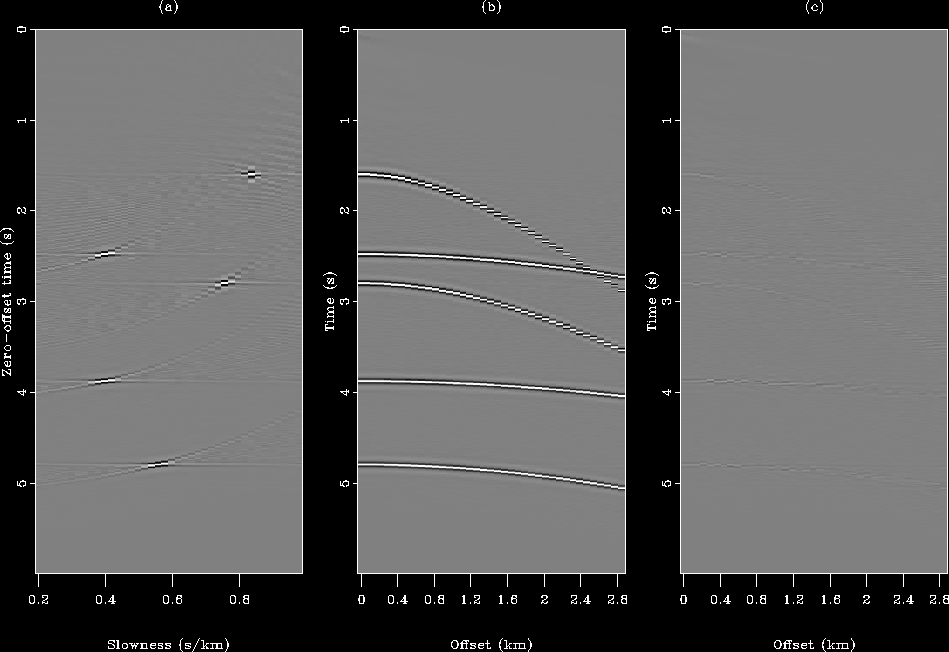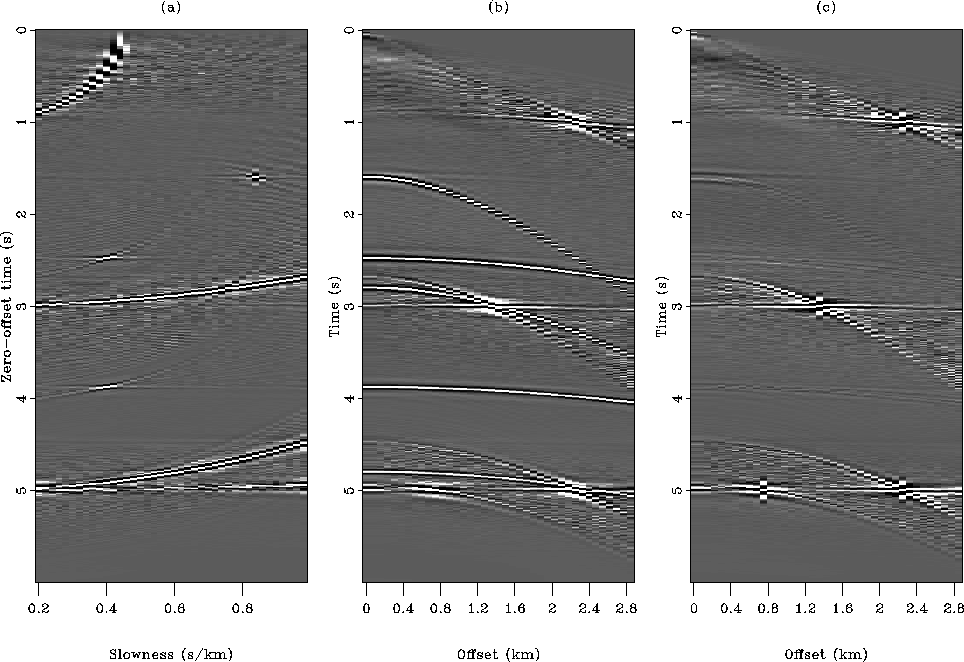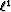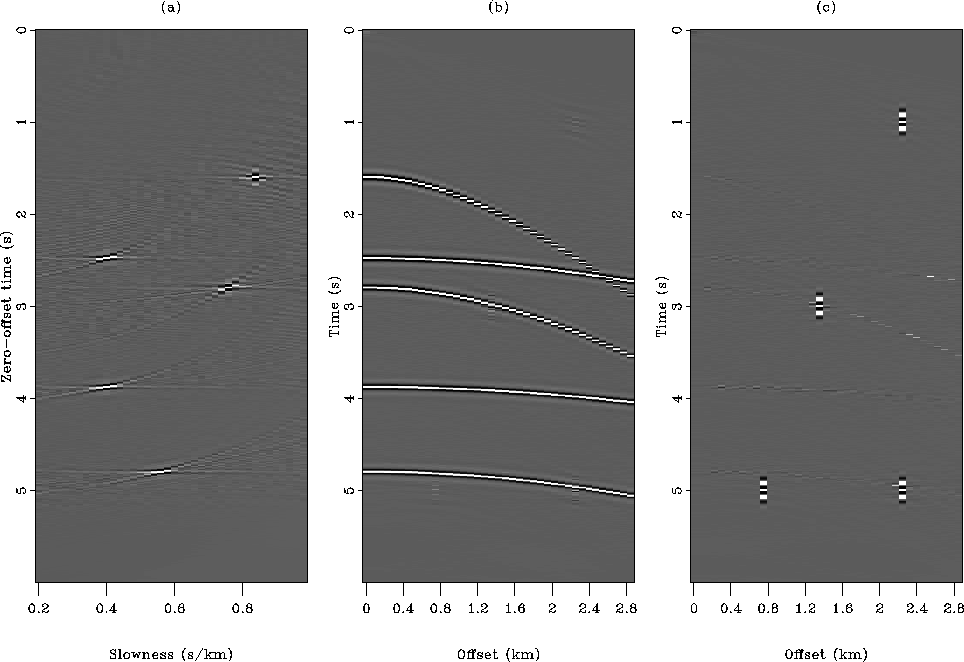Next: Field data results Up: Application of the Huber Previous: Application of the Huber

## Synthetic data results

Figuredisplays the synthetic model. In Figurea I show the ideal velocity space with five events at different slownesses. In Figureb the five corresponding hyperbolas in the CMP domain are shown. Finally in Figurec, four spikes are added to the CMP gather in Figureb to make gaussian statistics unsuitable. The energy of the four spikes is five times the energy of the five hyperbolas. Our goal is to find the velocity fieldthat will best fit a CMP gathervia the HRT.model
Figure 2
Synthetic data used for the inversion. (a) The true velocity model represented in slowness. (b) The noise-free input data used for the inversion. (c) The same data but with noise added (four spikes).Figureshows the result of the inversion when thenorm is used for the noise-free data. On the left the velocity space is displayed with its five events. The focusing is not perfect and some artifacts appear Sacchi and Ulrych (1995). The middle panel shows the remodeled data after inversion and the right panel displays the residual (difference between the input and remodeled data). I conclude that the inversion reached a minimum since no coherent energy is left in the residual: the data fitting is very good. Because the input data have gaussian statistics, the performance of the least-squares inversion was expected.res-nospike-HUBER
Figure 3
Result of the inversion with thenorm for the noise-free data. (a) Inverted slowness field. (b) Remodeled data. (c) Difference between the input (Figureb) and remodeled data.I now use the same inversion scheme with thenorm but with the contaminated'' CMP gather (Figurec). Notice that no regularization was applied with the least-squares method. The final result is shown in Figure. In this case, as expected, theinversion creates a number of artifacts both in the model and data space. In the model space (Figurea), the spikes are mapped into curves whereas the hyperbola are mapped into nearly focused points. In the data space, after remodeling (Figureb), spikes are not remodeled correctly and are smearing on the neighboring traces. In FigureI use least-squares with a simple damping in the regularization. The model and data space are cleaner but the difference between the input and the remodeled data or residual is still large (comparing Figurec to Figurec.) In addition, artifacts in the inverted slowness field and the reconstructed data can be seen.res-spike-L2-HUBER
Figure 4
Result of the inversion with thenorm for the data with noise. (a) Inverted slowness field. (b) Remodeled data. (c) Difference between the input (Figurec) and remodeled data. The four spikes create artifacts in both inverted model and reconstructed data space.res-spike-L2-reg-HUBER
Figure 5
Result of the inversion with thenorm with damping for the data with noise. (a) Inverted slowness field. (b) Remodeled data. (c) Difference between the input (Figurec) and remodeled data. The three panels are cleaner than in Figurebut some artifacts remain, however.Figuredisplays the result of the inversion with the Huber norm. The outcome of the inversion is insensitive to the spiky events, like a purenorm misfit function. The residual (Figurec) exhibits the four spikes very clearly. This result demonstrates that the proposed algorithm, although not specifically designed to minimize the Huber function, converges to a satisfying solution. The next section shows inversion results with noisy field data.res-spike-HUBER
Figure 6
Result of the robust inversion with the Huber norm for the data with noise. (a) Inverted slowness field. (b) Remodeled data. (c) Difference between the input (Figurec) and remodeled data. The Huber norm behaves like a purenorm since all artifacts have disappeared.Next: Field data results Up: Application of the Huber Previous: Application of the Huber
Stanford Exploration Project
5/5/2005